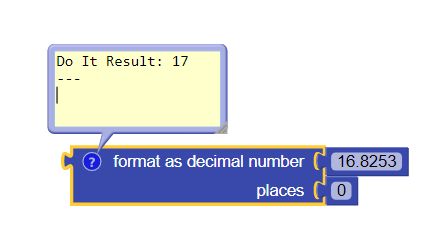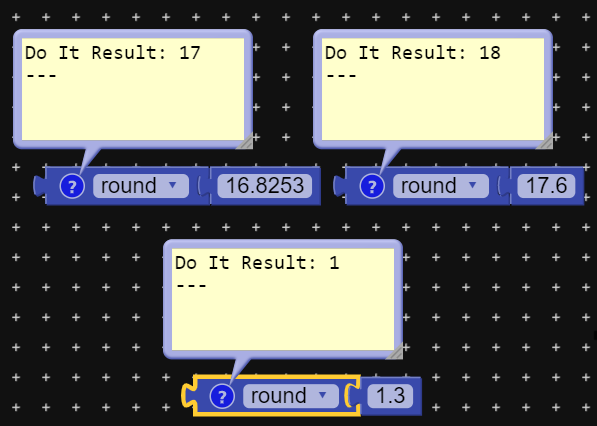# Decimal value to nearest number

I am getting a decimal value between 0 to 100 in multiple of 0.8 like 0.8 or 1.6 or 17.6 and so on.

I want this decimal value in nearest number in between 0 to 100.

For eg. If decimal value is 1.6 then i want 1 or 2

If decimal value is 17.6 then i want 17 or 18

Like this. I hope someone understand.
How to do this?

1 Like

Use format as decimal5 Likes

Suppose number = 9.6

There make if condition…
If( remainder of (number÷ 1)) > 0.5 then {
Set number to number + 1 - remainder of (number÷ 1)
}
Else{
Set number to **number - remainder of (number÷ 1)
}

So if decimal value is greater than .5 then it will move to succeeding number else it will preceed…

So according to above defined number it will give output 10

2 Likes

Thanks @dora_pazI am getting exactly what i want!

I am not much familiar with Math blocks.2 Likes

Out of curiosity, why not use the `round` block? I mean it will give the same result3 Likes

`round()` is a special case of `formatAsDecimal(x, y)` where y happens to be 0

2 Likes

As I said, I was not much familiar with the math blocks like format as decimal number block and round block.

Thanks for suggestion, but my problem is solved by using format as decimal number block.

@The_K_Studio

I just asked the question out of curiosity, you are free to use any method.@Vishwas
So, in simple words, for the method `format as decimal` , we have to assign ‘`0`’ to ‘y’ manually, whereas for `round` method it is automatically ‘`0`’. Am I right?

1 Like

Correct.

With `formatAsDecimal(x, y)`, you have the additional control over the number of decimal places.

if places set to 1 -> 1.27 will output 1.3; 1.24 will output 1.2
if places set to 2 -> 1.27 will output 1.27; 1.346 will output 1.35

But in you case `round` block should suffice.

3 Likes

the round block uses the round to even method, which probably is not what you like to use…
Taifun

see the documentation
https://docs.kodular.io/blocks/math/#round

Returns the given number rounded to the closest integer. If the fractional part is < .5 it will be rounded down. It it is > .5 it will be rounded up. If it is exactly equal to .5, numbers with an even whole part will be rounded down, and numbers with an odd whole part will be rounded up. (This method is called round to even .)

2 Likes

This topic was automatically closed 30 days after the last reply. New replies are no longer allowed.